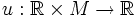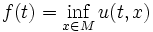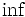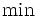# Timewise-min function

## Definition

Let$u:\R \times M \to \R$ be any continuous function. Then the timewise-min function of$u$ is a map$f:\R \to \R$ defined by:$f(t) = \inf_{x \in M} u(t,x)$

When$M$ is a compact manifold, we can replace$\inf$ by$\min$.